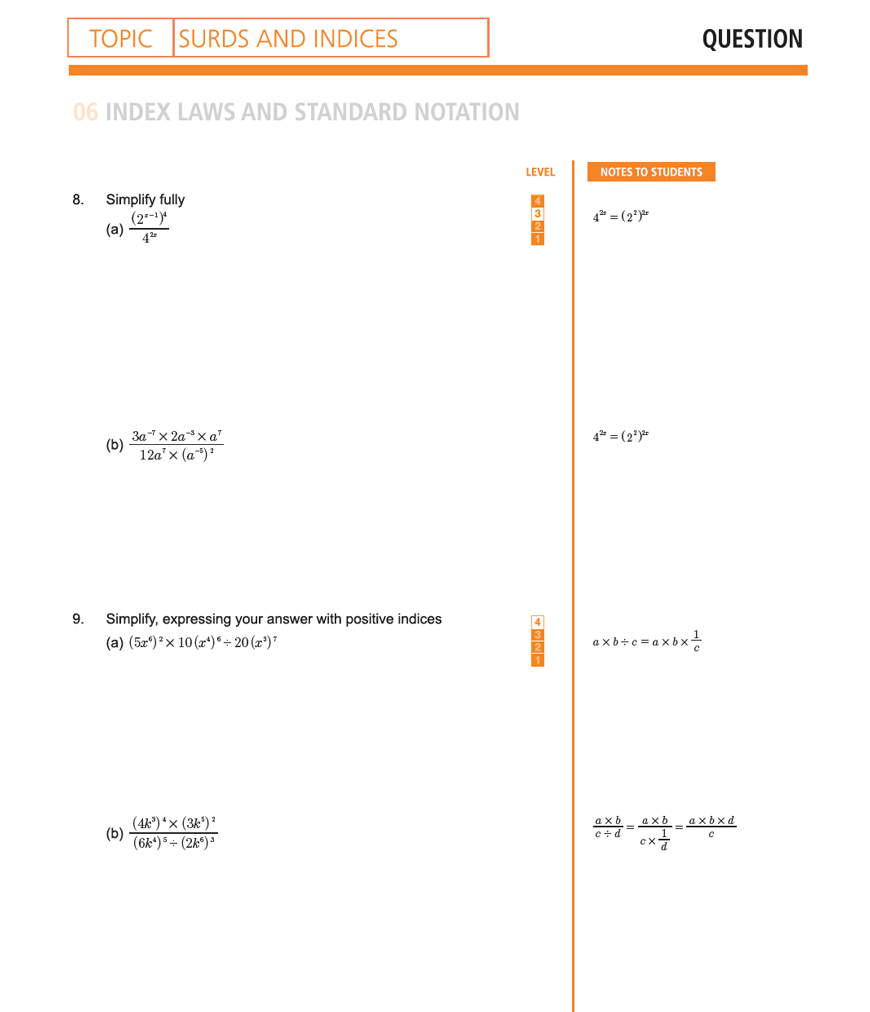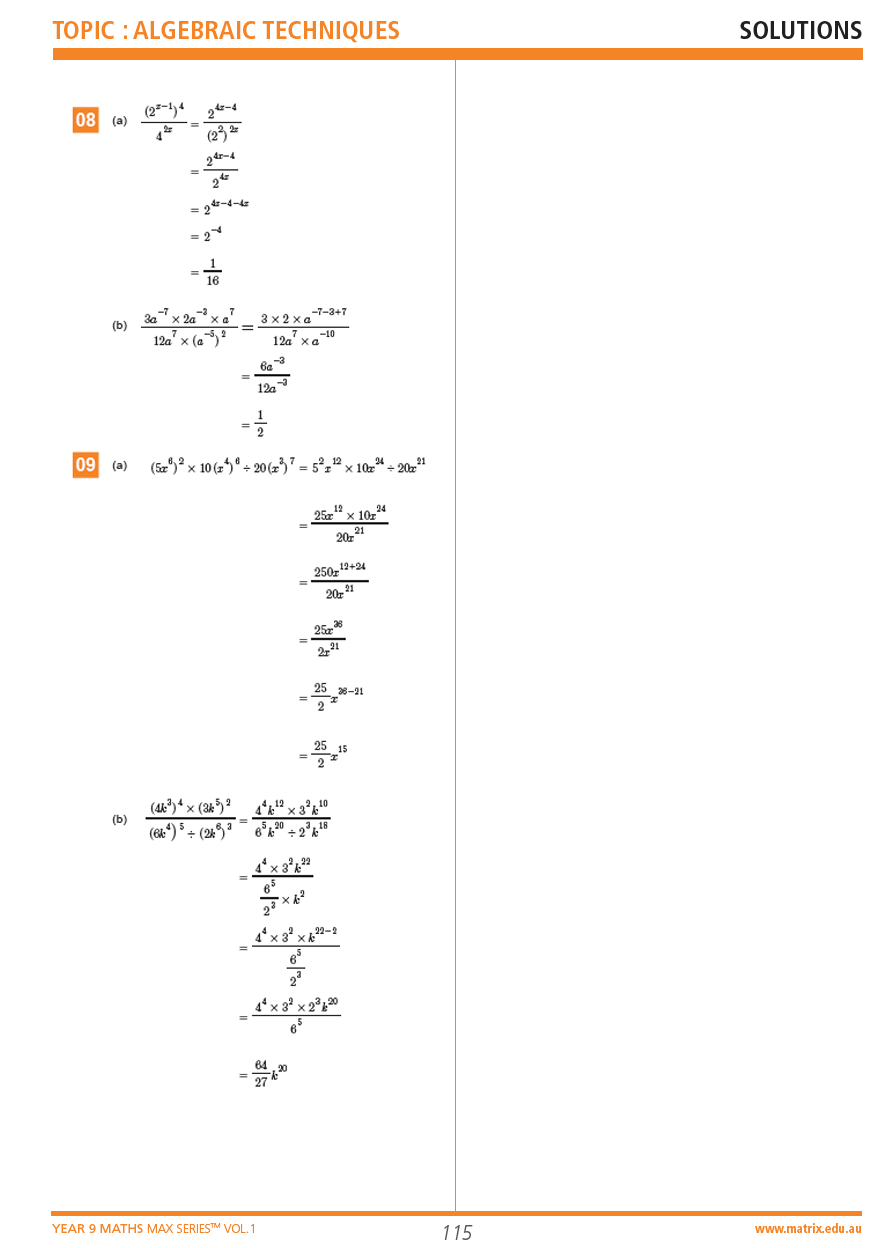# Part 3: Indices | Free Worksheet

In this article, we look at indices and surds and teach you how to use index notation.## Indices

In this article, we’re going to give you a rundown of what indices are and how to evaluate their operations, such as binomial products.

These are important subjects for Year 9 students. It is important that you have a thorough understanding of these concepts or you will really struggle in higher levels of Maths.

## Syllabus Outcome

Surds and Indices:

• Uses and interprets formal definitions and generalisations when explaining solutions and/or conjectures. MA5.3-1WM
• Generalises mathematical ideas and techniques to analyse and solve problems efficiently. MA5.3-2WM
• Uses deductive reasoning in presenting arguments and formal proofs. MA5.3-3WM
• Performs operations with surds and indices. MA5.3-6NA

This may seem like a long and convoluted set of statements. But what it is saying is really quite straightforward.

Essentially, these Outcomes state that in this unit you will learn that we have index laws for numbers and we have to adhere to these laws to solve problems.

This topic introduces you to operations involving indices.

You are expected to evaluate more complex expressions involving negative, zero and fractional indices, including simplification and binomial products.

They tend to become confused and forget the fundamentals of the indices topic when they get overwhelmed with a combination of different techniques.

Common mistakes include:

• Mixing up addition and multiplication of indices
• Confusing what to do when there are different bases as opposed to different indices
• Uncertainty around the role of brackets in expressions
• Incorrect simplification of more complex expressions

Manipulating indices can be challenging, especially when you have to combine multiple ideas from both topics to get the correct answer – however, not to worry!

This subject guide will point out common errors and help you better understand these new concepts.## Assumed knowledge

There is some prior knowledge needed before starting this topic.

You should have a grasp of basic indices – what does $$2^{3}$$ mean? What does $$3^{2}$$ mean? How are they different?

Additionally, you should be familiar with elementary BODMAS (Bracket, Of, Division, Multiplication, Addition and Subtraction) operations and simple algebraic expressions.

If you aren’t already comfortable with the concepts above, it’s a good idea to revise them before starting this topic – it will be extremely useful.

## Index notation and index laws

Index notation is a concise way of writing the repeated multiplication of the same factor. The factor is called the ‘base’ and the number of times it is repeated it called the ‘index’ or ‘power’.

eg. $$x^{4} \rightarrow x$$ is the base, and 4 is the index. It expands to $$x × x × x × x$$

There are special index laws that allow us to combine these sorts of expressions.

## Multiplication

When multiplying terms with the same base, indices are added.

$$x^m × x^n=x^{(m+n)}$$

You cannot multiply different bases in the same way to create a single base with the sum of indices.

So, $$x^{m} × y^{n} ≠xy^{(m+n)}$$

However, if they are different bases with the same power, the bases can then be multiplied. The power (or index) stays the same.

$$x^{m} × y^{m}= (xy)^{m}$$

### Examples

Let’s have a look at a few examples.

Example 1

$$y^6 × y^2$$

Solution 1

This question is quite simple; since they have the same base, $$y$$, the indices can be added to each other to get the answer $$y^8$$.

Example 2

$$a^2 × b × a^3 × b^4 × a^4$$

Solution 2

We are looking for terms with the same base. We see that there are 3 terms with base $$a$$, and 2 terms with base $$b$$.

This can essentially be rewritten as $$a^{2} \times a^{3} \times a^{4} \times b \times b^{4}$$  to get $$a^{2+3+4} \times b^{1+4}$$, which equals $$a^{9} \times b^{5}$$ (can also be written as $$a^9b^5$$)

Example 3

$$m^{x-5} \times 5m^{8-x}$$

Solution 3

The index here only applies to $$m$$­, so the 5 (coefficient) is treated separately.

In most cases, coefficients are added to the front of the other expressions.

$$5 \times m^{x-5} × m^{8-x} =5 \times m^{x-5+8-x}=5m^{3}$$

## Division

Division works quite similarly to multiplication. When dividing terms with the same base, the indices are subtracted from one another.

$$x^{m} \div x^{n} = x^{m-n}$$

When different bases with the same indices are divided, the bases are divided by each other.

$$x^{m} \div y^{m}=(\frac{x}{y})^m$$

### Examples

Have a go at some examples:

Example 1

$$10y^{9} \div 2y$$

Solution 1

As mentioned before, we treat the coefficients separately. This expression becomes

$$10 \div 2 \times y^{9} \div y \\ =5 \times y^{9-1} \\ =5y^{8} \\$$

Example 2
$$40h^{20} \div 5h^{3} \div 2h^{2}$$

Solution 2

Same method as above, but repeated once again.

Remember that we do not have to work on everything at the same time.

You should aim to work on each question step by step. It will be correct – as long as you follow the BODMAS operations.

$$8h^{20-3} \div 2h^{2} \\ =4h^{17} \div 2h^{2} \\ =2h^{15} \\$$

Example 3

$$30x^{11} y^{2} ÷2x^{3} × 4xy$$

Solution 3

Same method as above, but repeated once again.

Remember that we do not have to work on everything at the same time.

You should aim to work on each question step by step. It will be correct – as long as you follow the BODMAS operations.

$$30x^{11} y^{2} ÷2x^{3} × 4xy \\ = 15x^{8} y^{2} × 4xy \\ =60x^{9} y^{3} \\$$

## Power of a power

When you have a power of a power, you multiply the two indices together as such:

$$(x^{m})^{n}=x^{mn}$$

This is a common point of confusion among many students.

When you have an expression like this, you should remember to multiply the two powers together, instead of doing this:

$$(x^{m} )^{n} \neq x^{m^n}$$

Other important points to note:

$$(ax^{m})^{n}=a^{n} x^{mn}$$ $$a(x^{m} )^{n}=ax^{mn}$$ $$(\frac{x}{y})^{n}= \frac{x^{n}}{y^{n}}$$

### Examples

Let’s have a look at some examples:

Example 1

$$(2xy^{3})^{4}$$

Solution 1

All terms inside the bracket are raised to the power of 4;

$$(2xy^{3} )^{4} =2^{4}×x^{1×4}×y^{3×4} =2x^{4} y^{12}$$

Example 2

$$5(m^{2} n^{5} )^{3}$$

Solution 2

Here, only the terms inside the bracket are raised to the power of 3. The 5 stays as it is.

$$5×m^{2×3}×n^{5×3}=5m^{6} n^{15}$$

Example 3

$$(4x^{2} )^{3} × 2(x^{4} )^{2}$$

Solution 3

Every term in the first part is cubed, while the 2 is not squared in the second part. Therefore:

$$(4x^2 )^3 × 2(x^4 )^2 \\ =4^{3} x^{6} × 2×x^{8} (\text{combining numbers and pronumerals}) \\ =8x^{14}$$

## The Zero Index

When any expression is raised to the power of zero, the answer will always be 1.

$$x^{0}=1 (5x)^{0}=1 (6x+2)^{0}=1$$

But how about $$5x^{0}$$? This is different to the second example above. In this case, only $$x$$ is raised to the power of $$0$$.

So, using our knowledge of index operations, we know that $$5x^{0}=5×1=5$$

Try this one:

$$7^{0} × (2^{2} )^{0}$$

## Negative Indices

Negative indices essentially give you the reciprocal of the expression. Many questions will ask you to write expressions with positive indices. This means that you would have to use the properties below:

$$x^{-m}=\frac{1}{x^{m}} \\ ax^{-m}=\frac{a}{x^{m}} \\$$

If you have $$5x^{-2}$$, remember that the negative is only applied to x here. You would have to treat the number and the index expression separately $$\rightarrow 5 × x^{-2}$$, and combine them into one after reciprocating $$x \rightarrow 5× \frac{1}{x^{2}} = \frac{5}{x^{2}}$$

Try these:

1. $$\Big( \frac{2}{3} \Big) ^{-1}$$

2. $$5x^{-7}$$

You should get $$\frac{3}{2}$$ and $$\frac{5}{x^{7}}$$.

## Fractional indices

$$x^{\frac{1}{n}}= \sqrt[n]{x}$$

$$x^{\frac{m}{n}}= \sqrt[n]{x^{m}}$$

For fractional indices, the base is raised to the power of the numerator, and then rooted by the denominator. That is, if you have $$x^{\frac{2}{3}}$$, $$x$$ is first squared then put to the cube root.

$$x^{\frac{2}{3}}= \sqrt{x^{2}}$$

Take another example:

$$y^{\frac{-4}{5}}$$

This combines both negative and fractional indices together. If the question asks you to write it with positive integer indices, you would first deal with the negative:

$$y^{\frac{-4}{5}}=\frac{1}{y^{\frac{4}{5}}}$$

then:

$$\frac{1}{y^{\frac{4}{5}}} =\frac{1}{\sqrt{y^{4}}}$$

If you were to convert from surd form to index form,

$$\sqrt{x^{5}}=x^{\frac{5}{4}}$$

## Additional Challenging Examples (from the MAX Series)

### QuestionsQuestions from the Year 9 Maths Max Series Vol 1: Algebraic TechniquesAnswers from the Year 9 Maths Max Series Vol 1: Algebraic Techniques

## The Real Number System:

Real numbers are any numbers that can be represented on a number line. There is also something called an “imaginary” number, but you don’t need to worry about it. This is learned in more advanced maths later on!

The real number system can be divided into two subgroups: rational and irrational.

Rational numbers are those that can be written as a fraction, $$\frac{p}{q}$$, where $$p$$ and $$q$$ are whole numbers.

For example:

• Integers
• Fractions (proper, improper, mixed)
• Decimals (terminating and recurring)

Irrational numbers are, therefore, those that cannot be written as a fraction.

This includes:

• Roots (surds)
• Decimals that continue without terminating or repeating## Summary

These are the concepts we’ve covered in this guide:

## Indices:

### Multiplication

$$x^m × x^n=x^{m+n}$$
$$x^m × y^m= (xy)^m$$

### Division

$$x^m ÷ x^n=x^{(m-n)}$$
$$x^m ÷ y^m= \Big( \frac{x}{y} \Big) ^{m}$$

### Power of a power

$$(x^m )^n=x^{mn}$$

### The Zero Index

$$x^0=1$$

### Negative Indices

$$x^{-m}=\frac{1}{x^{m}}$$

### Fractional Indices

$$x^{\frac{m}{n}}= \sqrt[n]{x^{m}}$$

## Want to revise but running low on time?

Don’t worry!

In our 2-Day Year 9 Maths Holiday Course, we teach your key Maths concepts and help you build strong Maths foundations in just 2 days!© Matrix Education and www.matrix.edu.au, 2021. Unauthorised use and/or duplication of this material without express and written permission from this site’s author and/or owner is strictly prohibited. Excerpts and links may be used, provided that full and clear credit is given to Matrix Education and www.matrix.edu.au with appropriate and specific direction to the original content.